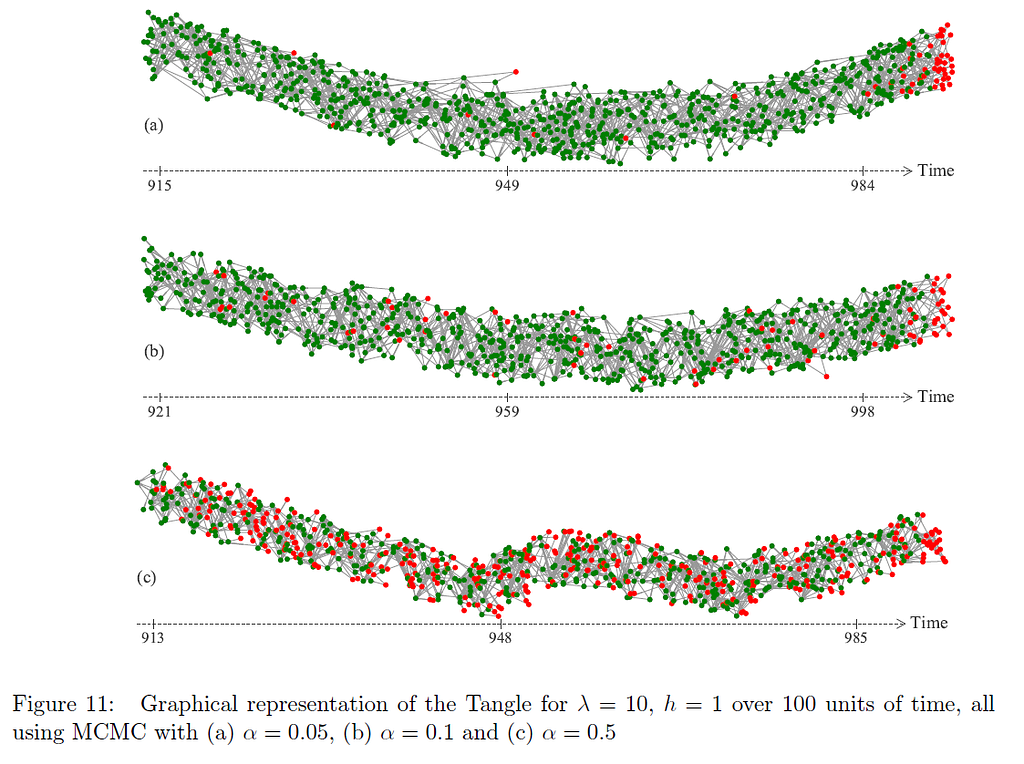# IOTA Research — Simulations

The IOTA Research team works intensively on the technology behind IOTA. We analyze the Tangle DLT (distributed ledger technology) using both analytical tools and numerical simulations. Recently, IOTA Researcher, Alon Gal, published a blog post about confirmation rates in the Tangle where he discussed the weighted-walk based consensus mechanism. Likewise, Professor Serguei Popov and his PhD students published an article about the equilibria in the Tangle. You can read the two part blog post explaining their findings here and here. Today, we publish this blog and accompanying research paper where we discuss results of numerical simulations of a continuous-time model of the Tangle.

This research paper largely confirms the analytical predictions of the original white paper. We demonstrated that the growth of the cumulative weight follows two stages: an adoption phase (which appears to be exponential), followed by a linear phase. These findings were independent of which tip selection algorithm was used; be it the MCMC random walk with some small α (α defines the randomness of the walk, where the lower the α the more random the walk is), or a selection of any random available tip (a uniform random tip selection). We also examined properties of Tangles where the number of directly approved transactions is greater than k=2. We confirmed that the ratio of average number of tips to the incoming transaction rate, λ, equals k/(k-1). Lastly, we analyzed the average total tip count tip count when k is a random variable.

You can read the full research paper here.In this image, the red vertices are tips (transactions which have not (yet) been approved).

IOTA Research — Simulations was originally published in IOTA on Medium, where people are continuing the conversation by highlighting and responding to this story.